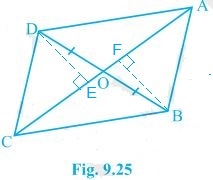Guru

# In Fig. 9.25, diagonals AC and BD of quadrilateral ABCD intersect at O such that OB = OD. If AB = CD, then show that:(iii) DA || CB or ABCD is a parallelogram. Q.6(3)

• 0

Please guide me the best way for solving the question of class 9th math of Areas of Parallelograms and Triangles chapter of exercise 9.3of math of question no.6(3) What is the best way for solving this question, please guide me

In Fig. 9.25, diagonals AC and BD of quadrilateral ABCD intersect at O such that OB = OD. If AB = CD, then show that:(iii) DA || CB or ABCD is a parallelogram.

Share

1. This answer was edited.Given,

(iii) When two triangles have same base and equal areas, the triangles will be in between the same parallel lines

ar(ΔDCB) = ar(ΔACB)

DA || BC — (iv)

For quadrilateral ABCD, one pair of opposite sides are equal (AB = CD) and other pair of opposite sides are parallel.

∴, ABCD is parallelogram.

• 0